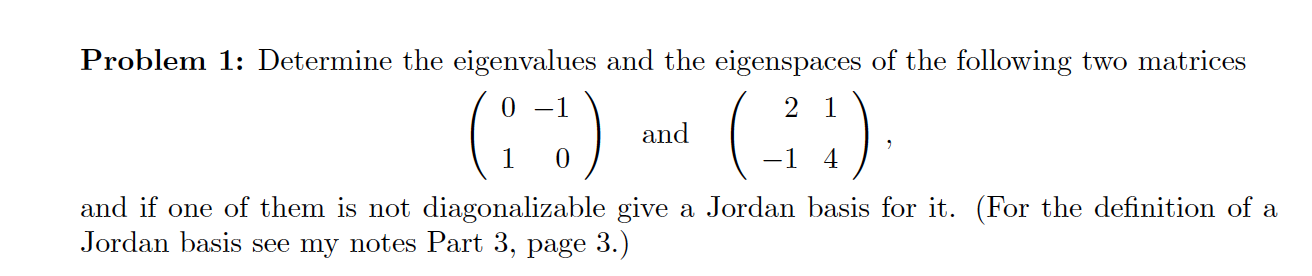Home / Answered Questions / Other / problem-1-determine-the-eigenvalues-and-the-eigenspaces-of-the-following-two-matrices-and-and-if-one-aw148

# (Solved): Problem 1: Determine The Eigenvalues And The Eigenspaces Of The Following Two Matrices ( -;) And (-)...Problem 1: Determine the eigenvalues and the eigenspaces of the following two matrices ( -;) and (-) and if one of them is not diagonalizable give a Jordan basis for it. (For the definition of a Jordan basis see my notes Part 3, page 3.)

We have an Answer from Expert